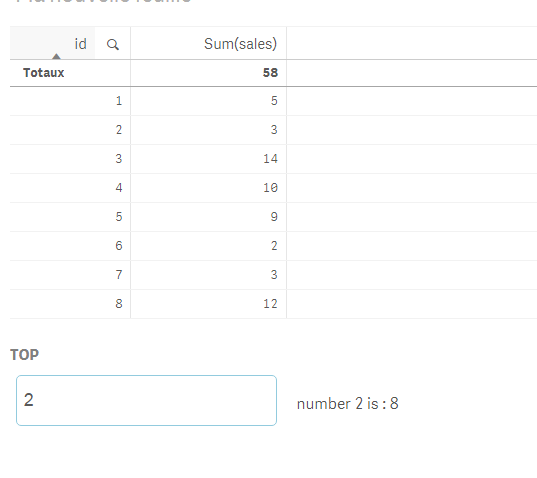Announcements
You can succeed best and quickest by helping others to succeed. Join the conversation.
cancel
Showing results for
Did you mean:Creator

## Pass Parameter into Qlik Function

Hi,

I want to define an eExpression, with a Parameter to deciced the "rank'" - so if I want the 1st or 2nd or 3rd  - sorted value:

=FirstSortedValue(Field, SortWeight, \$1)

The real expression is this, works ok if I use a number or nothing for the 3rd Param.

=FirstSortedValue( TOTAL   DIM1&';'&DIM2,
-Aggr(

\$(eZZZ) / \$(eYYY_0) +
\$(eZZZ) / \$(eYYY_1) +
\$(eZZZ) / \$(eYYY_2)
, DIM1,  DIM2)
,\$1
)

Thanks! Hope I can do this 🙂

3 RepliesMVP

Well you can add a variable maybe?

Let's call it : vParam :As an expression :

=' number '&(vParam)& ' is : '&FirstSortedValue(id, -sales, \$(vParam))Creator
Author

Thanks for your reply, it works ok, but I have a different use case.

I use the expression several times parallely, so it has to return DIM1&DIM2 value at the same time for the Rank No. 1, No. 2. and No. 3.

Any more ideas? I am not sure if the synthax of the 3rd parameter (\$1) is ok?

Thanks!Creator
Author

I made it working, the issue was not with the Parameter but the eExpression definition:

=FirstSortedValue(TOTAL Field, SortWeight, \$1) not OK,  if used in chart or textbox like: '\$(FirstSortedExpr(2))'

=FirstSortedValue(TOTAL Field, SortWeight, 2)  OK if used in chart or textbox like: '\$(FirstSortedExpr)'

FirstSortedValue(TOTAL Field, SortWeight, \$1) OK,  if used in chart or textbox like: \$(FirstSortedExpr(2))

So I assume it must be that in the above examples, the Expression was calculated 'in the background' (first) and its result delivered as a string.

In the 3rd, the Expression is only calculated in the front end object with the passed paramter.

Any feedback / affaimation on this would be appreciated...  Thanks!Tags
Community Browser